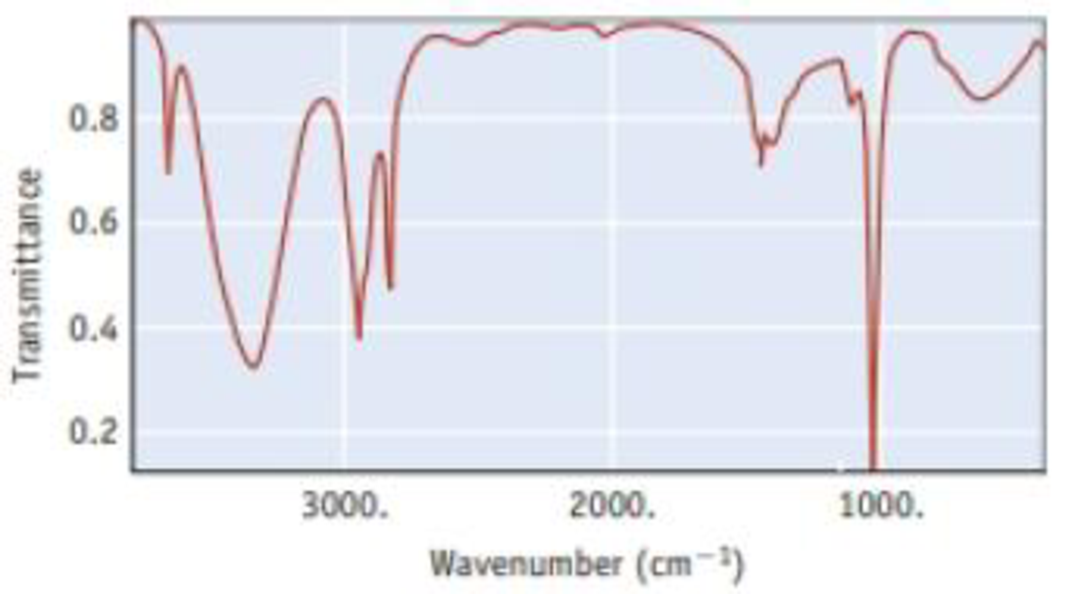Chapter 6, Problem 73IL

Chapter
Section
Textbook Problem

The infrared spectrum for methanol. CH3OH, is illustrated below. It shows the amount of light in the infrared region that methanol transmits as a function of wavelength. The vertical axis is the amount of light transmitted. At points near the top of the graph, most of the incident light is being transmitted by the sample (or, conversely, little light is absorbed). Therefore, the ″peaks″ or ″bands″ that descend from the top indicate light absorbed; the longer the band, the more light is being absorbed. The horizontal scale is in units of ″wavenumbers,″ abbreviated cm−1. The energy of light is given by Planck′s law as E = hc/λ; that is, E is proportional to 1/λ. Therefore, the horizontal scale is in units of 1/λ and reflects the energy of the light incident on the sample.(a) One point on the horizontal axis is marked as 2000 cm−1. What is the wavelength of light at this point? (b) Which is the low energy end of this spectrum (left or right), and which is the high energy end? (c) The broad absorption at about 3300-3400 cm−1 indicates that infrared radiation is interacting with the OH group of the methanol molecule. The narrower absorptions around 2800-3000 cm−1 are for interactions with C—H bonds. Which interaction requires more energy, with O—H or with C—H?

(a)

Interpretation Introduction

Interpretation: The wavelength of the light at the point 2000cm1 has to be determined

Concept introduction:

• The frequency of the light is inversely proportional to its wavelength.

ν=cλwhere, c=speedoflightν=frequencyλ=wavelength

• Wavelength and wavenumber are inversely proportional to each other.

Wavelength=1wavenumber

• Planck’s equation,

E=where, E=energyh=Planck'sconstantν=frequency

The energy increases as the wavelength of the light decrease. Also the energy increases as the frequency of the light increases.

Explanation

Given,

Wavenumber=2000cm1

The wavelength of the light can be calculated as,

Wavelength=1Wavenumber

Explanation

(b)

Interpretation Introduction

Interpretation: The high energy and low energy end of spectrum has to be determined.

Concept introduction:

• Wavelength and wavenumber are inversely proportional to each other.

Wavelength=1wavenumber

• Planck’s equation,

E==hcλwhere, E=energyh=Planck'sconstantν=frequency

The energy increases as the wavelength of the light decrease. Also the energy increases as the frequency of the light increases.

(c)

Interpretation Introduction

Interpretation: The interaction that requires more energy has to be determined among OH or CH.

Concept introduction:

• The frequency of the light is inversely proportional to its wavelength.

ν=cλwhere, c=speedoflightν=frequencyλ=wavelength

• Wavelength and wavenumber are inversely proportional to each other.

Wavelength=1wavenumber

• Planck’s equation,

E=where, E=energyh=Planck'sconstantν=frequency

The energy increases as the wavelength of the light decrease. Also the energy increases as the frequency of the light increases.

Still sussing out bartleby?

Check out a sample textbook solution.

See a sample solution

The Solution to Your Study Problems

Bartleby provides explanations to thousands of textbook problems written by our experts, many with advanced degrees!

Get Started

Find more solutions based on key concepts